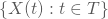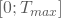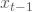# Which is the most interesting stochastic process

## Stochastic processes

If you have given a sequence of random variables X (t) that depend on a changing parameter t, you speak of a stochastic process. In general, t can run through any parameter space, but is often interpreted as time. This is why stochastic processes are also referred to as the dynamization of probability theory.

Formally, you describe a stochastic process asX (t) can, for example, represent the price of a share at time t within a time period T. You can then differentiate between the following situations and classify the stochastic processes accordingly:

• Discrete / continuous time observation
For example, if you are interested in the daily closing prices, you have given a market value X (t) for every day t. T then stands for a discrete set of points in time and the process is called discrete-time.
On the other hand, if T is an intervaland if you are interested in every value of the stock within this interval, you are talking about continuous processes.
• Discrete / continuous set of values
If you assume for your process that X (t) can only assume many different values ​​in a countable manner, the process is called discrete values; In the case of your share price, this is the case if you accept a cent as the smallest possible change. If, on the other hand, you assume a continuous course of X (t), in which the process can assume any value within an interval, then the process is called value-continuous.
• Stochastic dependencies
The stochastic dependencies of your process determine the expectations that you have for your process X (t) in t if you know the realizations up to (t-1). Then you can distinguish the following special processes:
• In the Markov process, the distribution of X (t) at time t is only dependent on its realization at time (t-1); all previous realizations are irrelevant. You can also speak of a "short memory" process.
• A martingale is a stochastic process in which the expected value of X (t) corresponds to its realization in (t-1):If X (t) is again your unknown share price at time t and isIf your market value in the previous period, the expected losses and increases in value will offset each other and you do not expect any changes on average. You can also speak of fair play in this context.

If the expected value of X (t) is greater than the known realization in (t-1), i.e. if you expect price gains, your price development is a submartingale; if your expected value in t is below the realization in (t-1), i.e. if you expect exchange rate losses, your process is called supermartingale.

In contrast to the time series analysis, which tries to explain the realizations of the X (t) through models, you consider the dynamic behavior of the random variables over time in the stochastic processes.